Test: Synchronous & Asynchronous Circuits

# Test: Synchronous & Asynchronous Circuits

Test Description

## 10 Questions MCQ Test GATE Computer Science Engineering(CSE) 2023 Mock Test Series | Test: Synchronous & Asynchronous Circuits

Test: Synchronous & Asynchronous Circuits for Computer Science Engineering (CSE) 2023 is part of GATE Computer Science Engineering(CSE) 2023 Mock Test Series preparation. The Test: Synchronous & Asynchronous Circuits questions and answers have been prepared according to the Computer Science Engineering (CSE) exam syllabus.The Test: Synchronous & Asynchronous Circuits MCQs are made for Computer Science Engineering (CSE) 2023 Exam. Find important definitions, questions, notes, meanings, examples, exercises, MCQs and online tests for Test: Synchronous & Asynchronous Circuits below.
Solutions of Test: Synchronous & Asynchronous Circuits questions in English are available as part of our GATE Computer Science Engineering(CSE) 2023 Mock Test Series for Computer Science Engineering (CSE) & Test: Synchronous & Asynchronous Circuits solutions in Hindi for GATE Computer Science Engineering(CSE) 2023 Mock Test Series course. Download more important topics, notes, lectures and mock test series for Computer Science Engineering (CSE) Exam by signing up for free. Attempt Test: Synchronous & Asynchronous Circuits | 10 questions in 30 minutes | Mock test for Computer Science Engineering (CSE) preparation | Free important questions MCQ to study GATE Computer Science Engineering(CSE) 2023 Mock Test Series for Computer Science Engineering (CSE) Exam | Download free PDF with solutions
 1 Crore+ students have signed up on EduRev. Have you?
Test: Synchronous & Asynchronous Circuits - Question 1

###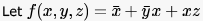be a switching function. Which one of the following is valid?

Detailed Solution for Test: Synchronous & Asynchronous Circuits - Question 1

f(x,y,z) = x' + y'x + xz
An implicant of a function is a product term that is included in the function. so x', y'x and xz ,all are implicants of given function.
A prime implicant of a function is an implicant that is not included in any other implicant of the function.
option a)   y'x is not a prime implicant as it is included in xz [ xy'z+ xyz]
option d) y is not a prime implicant as it include in both x' and xz.
a product term in which all the variables appear is called a minterm of the function

Test: Synchronous & Asynchronous Circuits - Question 2

### Which are the essential prime implicants of the following Boolean function?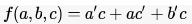Detailed Solution for Test: Synchronous & Asynchronous Circuits - Question 2

using K map f = ac' + a'c

Test: Synchronous & Asynchronous Circuits - Question 3

### A main memory unit with a capacity of 4 megabytes is built using 1M x 1-bit DRAM chips. Each DRAM chip has 1K rows of cells with 1K cells in each row. The time taken for a single refresh operation is 100 nanoseconds. The time required to perform one refresh operation on all the cells in the memory unit is

Detailed Solution for Test: Synchronous & Asynchronous Circuits - Question 3

There are 4*8 = 32 DRAM chips to get 4MB from 1M x 1-bit chips. Now, all chips can be refreshed in parallel so do all cells in a row. So, the total time for refresh will be number of rows times the refresh time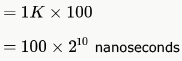*Answer can only contain numeric values
Test: Synchronous & Asynchronous Circuits - Question 4

A ROM is used to store the Truth table for a binary multiple unit that will multiply two 4-bit numbers. The size of the ROM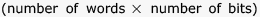that is required to accommodate the Truth table is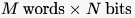. Write the values of M and N.

Detailed Solution for Test: Synchronous & Asynchronous Circuits - Question 4

A is 4 bit binary no A4A3A2A1
B is 4 bit binary no B4B3B2B1
M is result of multiplication M8M7M6M5M4M3M2M1     [check biggest no 1111 x 1111 =11100001]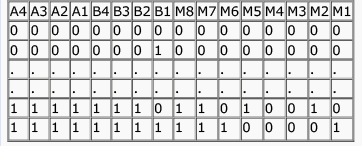So 4 bit of A 4 bit of B
input will consist of 8 bit need address 00000000 to 11111111 = 28 address
output will be of 8 bits
so memory will be of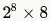M = 256 , N = 8

Test: Synchronous & Asynchronous Circuits - Question 5

A ROM is used to store the table for multiplication of two 8-bit unsigned integers. The size of ROM required is

Detailed Solution for Test: Synchronous & Asynchronous Circuits - Question 5

When we multiply two 8 bit numbers result can go up to 16 bits. So, we need 16 bits for each of the multiplication result.
Number of results possible = 28 ⨯ 28 = 216 = 64 K as we need to store all possible results of multiplying two 8 bit numbers. So, 64 K ⨯ 16 is the answer.

Test: Synchronous & Asynchronous Circuits - Question 6

What is the minimum size of ROM required to store the complete truth table of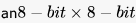multiplier?

Detailed Solution for Test: Synchronous & Asynchronous Circuits - Question 6

multiplying 2 8 bit digits will give result in maximum 16 bits
total number of multiplications possible = 28 x 28
hence space required = 64K x 16 bits

Test: Synchronous & Asynchronous Circuits - Question 7

The amount of ROM needed to implement a 4 - bit  multiplier is

Detailed Solution for Test: Synchronous & Asynchronous Circuits - Question 7

A ROM cannot be written. So, to implement a 4 bit multiplier we must store all the possible combinations of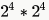input bits and  output bits giving a total of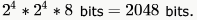. So, (D) is the answer.

Test: Synchronous & Asynchronous Circuits - Question 8

Which of the following input sequences for a cross-coupled R-S flip-flop realized with two NAND gates may lead to an oscillation?

Detailed Solution for Test: Synchronous & Asynchronous Circuits - Question 8

For R-S flip flop with NAND gates (inputs are active low) 11-no change 00-indeterminate..............so option A may make the system oscillate as "00" is the final input. In option D, after "00" flipflop output may oscillate but after "11", it will be stabilized.

Test: Synchronous & Asynchronous Circuits - Question 9

The below figure shows four D-type flip-flops connnected as a shift register using an XOR gate. The initial state and three subsequent states for three clock pulses are also given.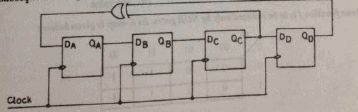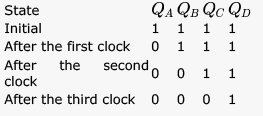The state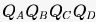after the fourth clock pulse is

Detailed Solution for Test: Synchronous & Asynchronous Circuits - Question 9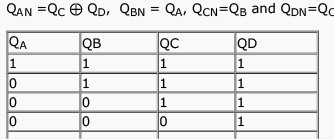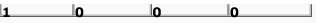Test: Synchronous & Asynchronous Circuits - Question 10

Consider the circuit given below with initial state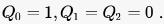. The state of the circuit is given by the value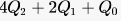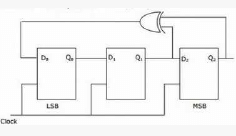Which one of the following is correct state sequence of the circuit?

Detailed Solution for Test: Synchronous & Asynchronous Circuits - Question 10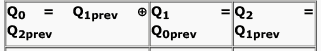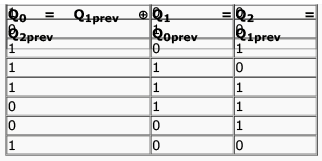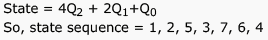## GATE Computer Science Engineering(CSE) 2023 Mock Test Series

150 docs|215 tests
 Use Code STAYHOME200 and get INR 200 additional OFF Use Coupon Code
Information about Test: Synchronous & Asynchronous Circuits Page
In this test you can find the Exam questions for Test: Synchronous & Asynchronous Circuits solved & explained in the simplest way possible. Besides giving Questions and answers for Test: Synchronous & Asynchronous Circuits , EduRev gives you an ample number of Online tests for practice

## GATE Computer Science Engineering(CSE) 2023 Mock Test Series

150 docs|215 tests